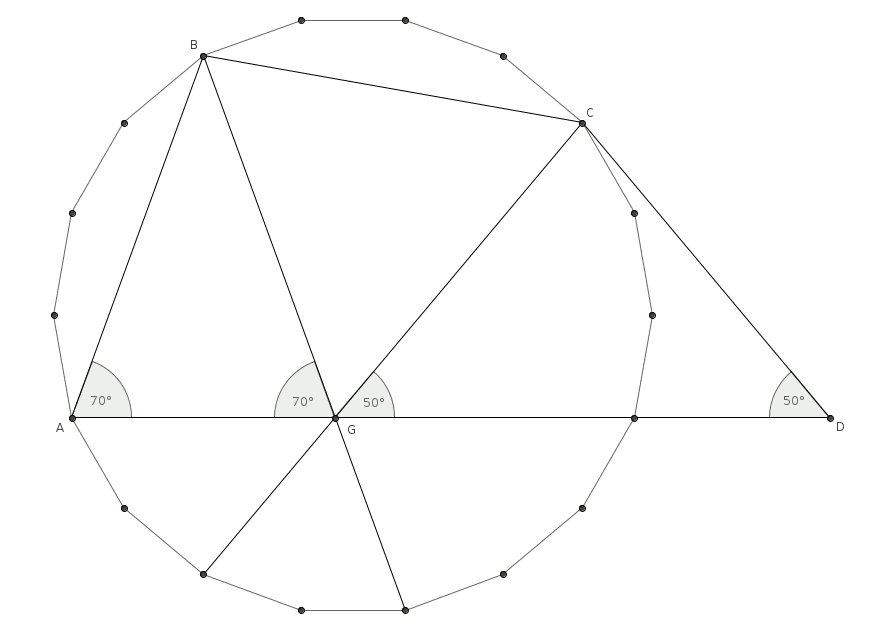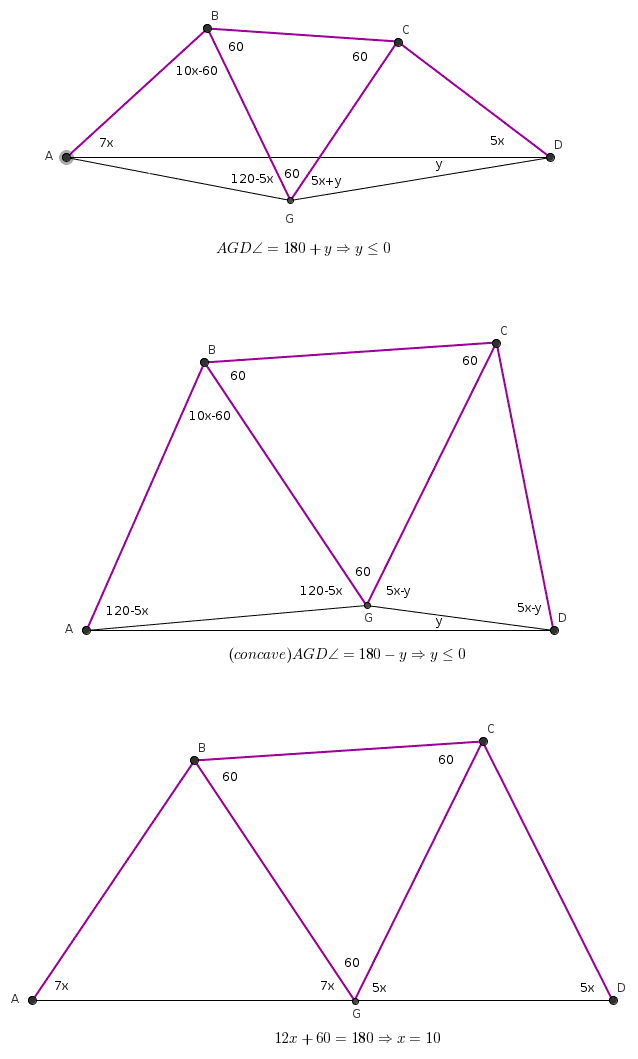Középiskolai Matematikai és Fizikai Lapok
Informatika rovattal
  Peter Erben 2022-01-14 11:20:32The motivation for the previous solution was a property of the diagonals of a regular 18-gon: their angle is always a multiple of $\displaystyle 10 ^\circ$. Also, we can choose three of these diagonals in many ways so that the three chosen diagonals are concurrent.Előzmény:  Peter Erben, 2022-01-14 11:08:41
  Peter Erben 2022-01-14 11:08:41Nice solution. Another approach could be to draw a $\displaystyle BCG$ equilateral triangle and prove by contradiction that $\displaystyle G$ should line on the segment $\displaystyle AD$.Előzmény:  Lpont, 2022-01-13 22:53:04
  Lpont 2022-01-13 22:53:04The bisector of section AC intersects section AD at E, and the bisector of section BD intersects section AD at F (ABCD is a convex quadrilateral). Due to AB=BC=CD, the ABCE and the BCDF quadrilaterals are deltoids, FBC angle is 5x and EBC angle is also 10x/2=5x. E is the same F because both points lie on the segment AD. Hereinafter, the notation E is used. Then AEB angle = BEC angle = CED angle = 60, hence 7x + 5x = 12x in the ABE triangle = 120. There is x = 10 and in the convex quadrilateral ABCD the angle at C is 360-22x = 140. The triangles ABE, BEC, and CED are congruent, with angles of 50, 60, and 70, respectively. Előzmény:  perash, 2022-01-13 19:36:30
  perash 2022-01-13 19:36:30yes but how
  Lpont 2022-01-13 09:37:02140, x=10 Előzmény:  perash, 2022-01-11 21:22:59
  perash 2022-01-11 21:22:59Given a convex quadrilateral $\displaystyle ABCD$ with $\displaystyle AB = BC = CD$ and $\displaystyle m(\angle DAB) = {7x }$,$\displaystyle \,m(\angle A BC) = {10x }$, $\displaystyle m(\angle CDA) = { 5x }$, Find $\displaystyle m(\angle BCD)$.